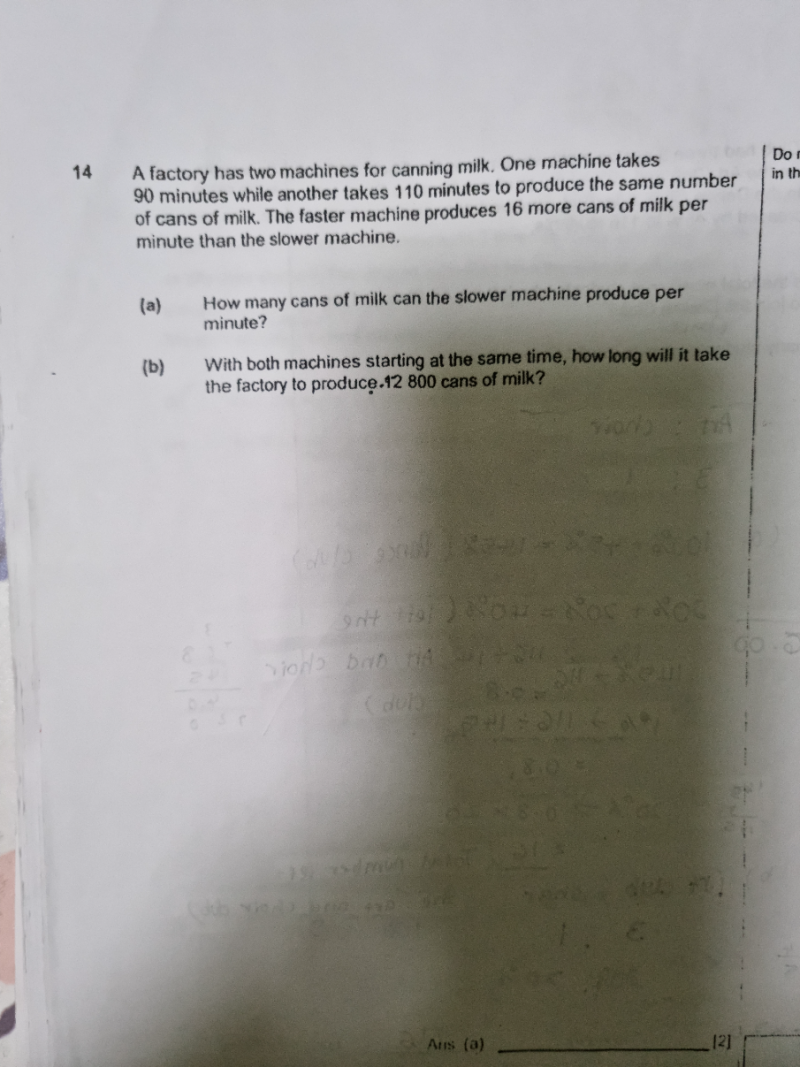# QuestionThank you.

(a)   90 min —> u   cans => 1 min = u/90    => 1 min = 7920/ 90  = 88

110 min —> u  cans  => 1 min = u/110    => 1 min = 7920/110  = 72

u/90 = (u/110) + 16    <—– x 990 on both sides,

11u = 9u + 15840

2u = 15840  => u = 15840/2 = 7920

substitute u =7920 into above  1 min rate.

Hence slower machine can produce 72 cans in 1 min.

(b)  88+72 = 160 cans

160 cans  –>  1 min

12800 cans –> 12800/160 = 80 mins

Hence both machines will take 1 hour 20 mins to produce 12800 cans.